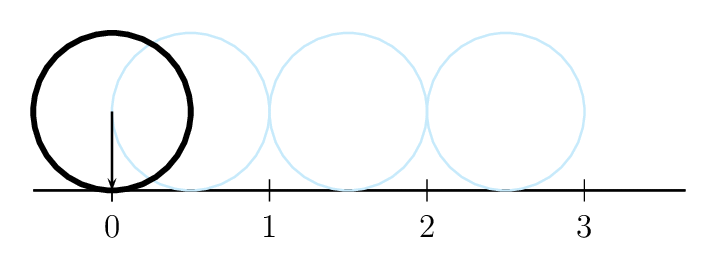# Rolling without Slipping of Rings, Cylinders and Spheres

Consider motion of a ball on a surface (fixed or moving). At the contact point, the ball may or may not slide on the surface. If tangential velocity of the ball at the contact point is equal to the velocity of the surface at this point then there is no slipping. Same is true for the acceleration.## Solved Problems from IIT JEE

### Problem from IIT JEE 2002

A cylinder rolls up an inclined plane, reaches some height and then rolls down (without slipping throughout these motions). The directions of the frictional force acting on the cylinder are,

1. up the incline while ascending and down the incline while descending.
2. up the incline while ascending as well as descending.
3. down the incline while ascending and up the incline while descending.
4. down the incline while ascending as well as descending.

Solution: The force acting on the cylinder are weight $mg$ and normal reaction $N$.In the absence of frictional force, the component $mg\sin\theta$ will give an acceleration $g\sin\theta$ down the incline and hence contact point will have a tendency to move downward. To oppose this tendency, the frictional force will act upward. This is independent of whether cylinder is moving up or down.

I encourage you to show that friction reduces the acceleration to $g\sin\theta/\left(1+\frac{I}{mR^2}\right)$.

### Problem from IIT JEE 2012

Two solid cylinders P and Q of same mass and same radius start rolling down a fixed inclined plane from the same height at the same time. Cylinder P has most of its mass concentrated near its surface, while Q has most of its mass concentrated near the axis. Which statement(s) is (are) correct?

1. Both cylinders P and Q reach the ground at the same time.
2. Cylinder P has larger linear acceleration than cylinder Q.
3. Both cylinders reach the ground with same translational kinetic energy.
4. Cylinder Q reaches the ground with larger angular speed.

Solution: Since cylinder P has most of its mass concentrated near its surface, its moment of inertia (about the cylinder axis) is more than the moment of inertia of the cylinder Q i.e., $I_P>I_Q$. The forces acting on the cylinder are its weight $mg$, normal reaction $N$, and frictional force $f$.In case of rolling without slipping, $v=\omega r$ and $a=\alpha r$. The torque about centre of mass is related to $\alpha$ by \begin{align} \label{wca:eqn:1} \tau=rf=I\alpha, \end{align} and the force along the plane is related to $a$ by \begin{align} \label{wca:eqn:2} mg\sin\theta-f=ma. \end{align} Solve the above equations to get \begin{align} \label{wca:eqn:3} a=\frac{g\sin\theta}{1+I/(mr^2)}. \end{align} The above equation gives $a_P < a_Q$ (since $I_P > I_Q$). Hence, P reaches the ground latter, it will have a lesser velocity, lesser angular velocity ($\omega=v/r$) and lesser translational kinetic energy. I encourage you to show same results by using the conservation of energy. Hint: $mgh=\frac{1}{2}mv^2+\frac{1}{2}I\omega^2$ gives $\frac{1}{2}mv_{P}^2 < \frac{1}{2}mv_{Q}^2$ and thus $v_{P} < v_Q$, $\omega_{P} < \omega_Q$, $a_{P} < a_Q$ and $t_{P} > t_Q$.

### Problem from IIT JEE 2007

A small object of uniform density rolls up a curved surface with an initial velocity $v$. It reaches up to a maximum height of $\frac{3v^2}{4g}$ with respect to the initial position. The object is,

1. ring
2. solid sphere
3. hollow sphere
4. discSolution: Let mass of the object be $m$, its radius be $r$, and moment of inertia about axis of rotation be $I$. We assume object to roll without slipping which gives, \begin{align} v=\omega r. \end{align} In rolling without slipping, work done by frictional force is zero and hence mechanical energy is conserved. Initial and final values of mechanical energy are, \begin{align} K_i+U_i&=K_i+0\\ &=\tfrac{1}{2}mv^2+\tfrac{1}{2}I\omega^2\\ &=\tfrac{1}{2}mv^2\left(1+\frac{I}{mr^2}\right),\\\ K_f+U_f&=0+U_f=mgh\\ &=mg\left(\frac{3v^2}{4g}\right)\\ &=\frac{3}{4}mv^2. \end{align} Equate initial and final mechanical energies to get $I=\frac{1}{2}mr^2$. This is moment of inertia of a disc about a normal axis passing through its centre.

### True or False Type Question from IIT JEE 1989

A ring of mass 0.3 kg and radius 0.1 m and a solid cylinder of mass 0.4 kg and of the same radius are given the same kinetic energy and released simultaneously on a flat horizontal surface such that they begin to roll as soon as released towards a wall which is at the same distance from the ring and the cylinder. The rolling friction in both cases is negligible. The cylinder will reach the wall first.

Solution: The time to reach the wall depends on the velocity of centre of mass. In pure rolling, the velocity of centre of mass and the angular velocity are related by $v=\omega r$, where $r$ is the distance of centre of mass from the contact point on the ground ($r$ is the radius of the ring/cylinder in the given case). Also, in pure rolling, there is no energy loss due to friction because point of contact does not move.

The kinetic energies (translational+rotational) of the ring and the cylinder are given by \begin{align} \label{uob:eqn:1} K_r&=\tfrac{1}{2}m_r v_r^2+\tfrac{1}{2}I_r \omega_r^2\nonumber\\ &=\tfrac{1}{2}m_r v_r^2+\tfrac{1}{2}(m_r r^2) (v_r/r)^2 \\ &=m_r v_r^2,\\ \label{uob:eqn:2} K_c&=\tfrac{1}{2}m_c v_c^2+\tfrac{1}{2}I_c \omega_c^2\nonumber\\ &=\tfrac{1}{2}m_c v_c^2+\tfrac{1}{2}\left(\tfrac{1}{2}m_c r^2\right) (v_c/r)^2 \\ &=\tfrac{3}{4}m_c v_c^2. \end{align} Given, kinetic energy of the ring and the cylinder are equal i.e., $K_r=K_t$. Substitute $K_r$ and $K_t$ from the first two equations to get \begin{align} %\label{uob:eqn:3} \frac{v_r}{v_c}&=\sqrt{\frac{3m_c}{4m_r}}=1. \end{align} Thus, velocities of the centre of mass of the ring and the cylinder are equal. Hence, both reach the wall simultaneously.